# Determine the Fourier transform of each of the following signals:1. x(t) =2. 1(t - 3) + 1(t + 3)3. 41 (4) cos(27 fot)4. t sinct

Question
3 views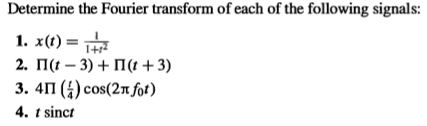help_outlineImage TranscriptioncloseDetermine the Fourier transform of each of the following signals: 1. x(t) = 2. 1(t - 3) + 1(t + 3) 3. 41 (4) cos(27 fot) 4. t sinct fullscreen
check_circle

(2.)rectangular function definition

the rectangular function is given by the expression as shown below:

the Fourier transform for the given expression is given as :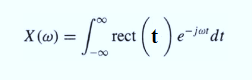Here in the given problem, the shifting property is used. the variable t shifts to (t-3).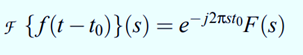shifting property for rectangular function.

the Fourier transform of the rectangular function is given by:

F(rect(t))=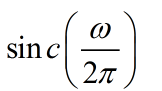applying the shifting property for the given function,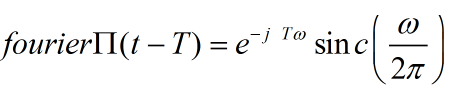calculation

the given problem can be broken into two parts by using Fourier distributive property. as shown below.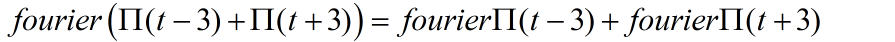calculation for the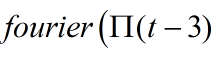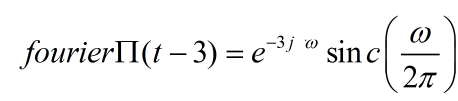The overall Fourier transform is given as...

### Want to see the full answer?

See Solution

#### Want to see this answer and more?

Solutions are written by subject experts who are available 24/7. Questions are typically answered within 1 hour.*

See Solution
*Response times may vary by subject and question.
Tagged in

### Electrical Engineering